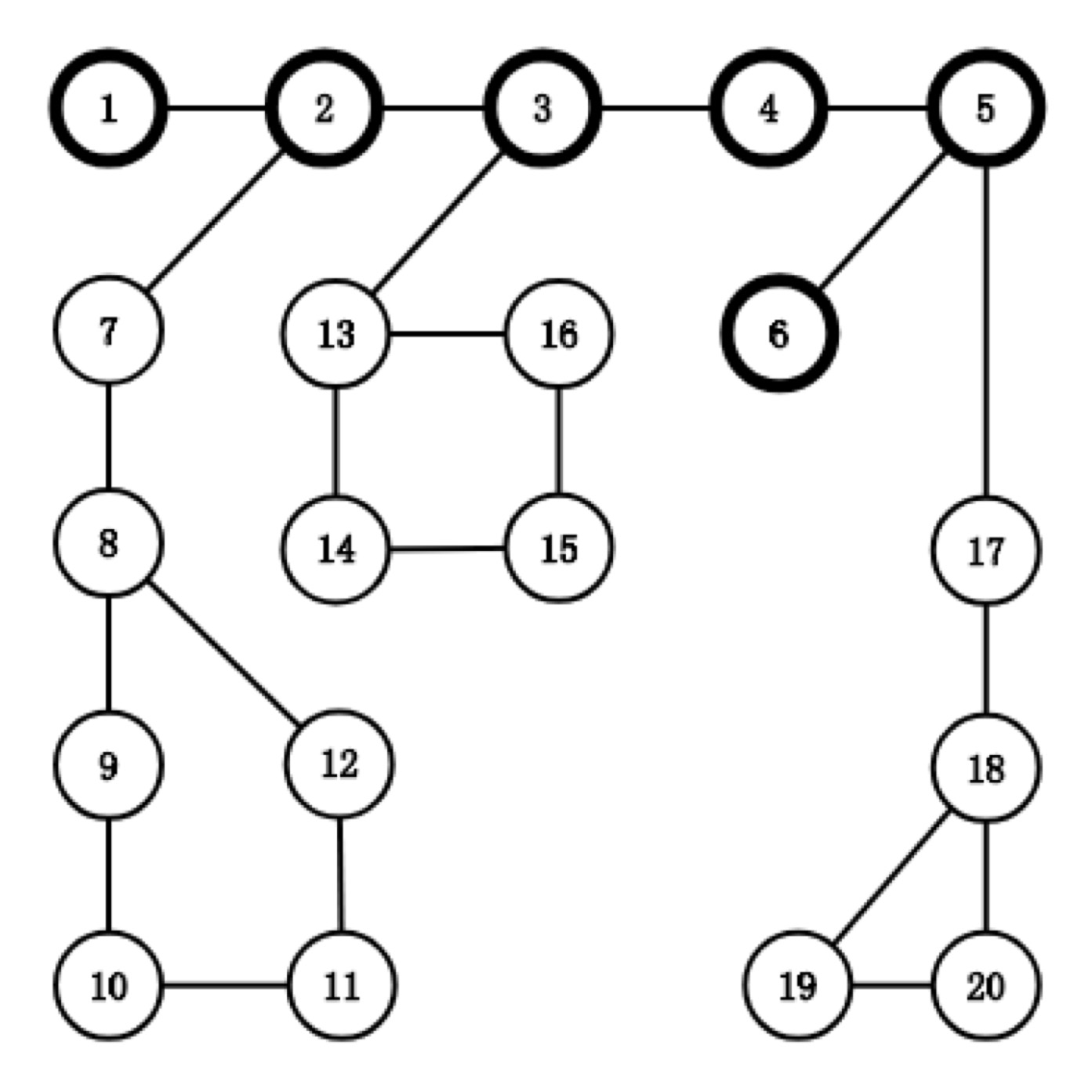시간 제한메모리 제한제출정답맞힌 사람정답 비율
1.5 초 (추가 시간 없음) 256 MB111100.000%

## 문제

An ordinary chain is a graph consisting of sequential (at least two) vertices. Every two adjacent vertices are connected by an edge. The $k$-th order Chiaki Chain looks slightly different from a chain. There are $k$ sub-chains of various lengths extended from $k$ different vertices on the main chain. At the other side of each sub-chain, there is a simple cycle of length $3, 4, \ldots, k + 2$ respectively. There is no useless vertices or edges on the $k$-th order Chiaki Chain.

Note that the main chain and the sub-chains should consist of at least two vertices.

The following image corresponds to the a $3$-rd order Chiaki Chain with $20$ vertices and $22$ edges:Given $n$, $m$ and $k$, Chiaki would like to know the number of labelled $k$-th order Chiaki Chain with $n$ vertices and $m$ edges. Since this number may be very large, you are only asked to calculate it modulo $10^9+7$.

## 입력

There are multiple test cases. The first line of the input contains an integer $T$ ($1 \le T \le 10^5$), indicating the number of test cases. For each test case:

The first line contains three integers $n$, $m$ and $k$ ($1 \le n, m, k \le 10^6$) --- the number of vertices and the number of edges in the graph and the order of Chiaki Chain.

## 출력

For each test case, output an integer denoting the answer.

## 예제 입력 1

4
1 1 1
3 3 1
4 4 1
5 5 1


## 예제 출력 1

0
0
0
60